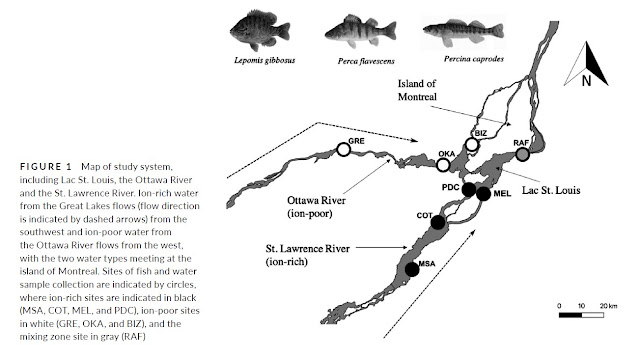## Sunday, December 4, 2022

### Within, among, or between?

I was recently surprised to learn from my students (I really appreciate that they spoke up about it) that some phrases I had been using were confusing - most specifically among-population variation.* This led to a discussion of the meaning of within, among, and between and how these terms are used in ecology and evolution (a microcosm of how they are used more generally). As the confusion appears to be more common than I thought, perhaps it is worth explaining the situation here.

To make this explanation clear, first imagine that you are analyzing a number of separate populations (e.g., humans in different populations) and that you have measured a particular trait (let's say body size) in a number of individuals in each of those populations. Note: this is not a random example, it is precisely what we did in a paper some years ago - McKellar et al. (2009). Below is a figure from that paper providing compilation of within-population variation (y-axis) and among-population variation (x-axis) measures (here the "coefficient of variation" - CV) within a large number of animal populations.

Within

If you report a descriptive statistic for each population separately, those measures are within-population summary statistics. Thus, within-population variation is a measure of variation within each of those populations - as might be indexed in a variance or standard deviation or coefficient of variation of body size for each of those populations separately. You can then also calculate the average or variation (across populations) of those within-populations measurements. In this case, you use the various within-population measures you have calculated (e.g., the variance within each population) as data to calculate another set of descriptive statistics, such as the mean (across populations) of the within-population variance.

I should note that, in some cases, one wishes to assume that these within-population measures are all estimating the same global (that is, shared across populations) within-population variance (or mean or whatever). In such cases, it can be assumed that populations with larger sample sizes (more individuals measured) are providing better estimates of that shared (common across populations) within-population parameter - and so the estimated average (across populations) of the within-population parameter is calculated by weighting the within-population estimates by their sample sizes. This is precisely what is done when one calculates a "pooled standard deviation." Of course, variation among the within-population estimates of the parameter are a measure of how much variation might exist among populations in those within-population parameters.

Between and Among

If you next report a descriptive statistic that examines trait variation across the populations, then you are in the world of between-population (if across only two of the populations) or among-population (if across three or more populations) estimates.** Typically, these estimates do NOT include variation within those populations. That is, you don't simply pool all of the individuals across all of your populations and calculate a single mean or variance - because this approach mixes within and among population variation.***

So, instead, the simplest approach is to take the within-population parameter estimates, such as the within-population means and variances of trait values for each of populations you measured, and use them as data points to calculate a new mean and variance. The first of these (the mean of the means) was mentioned above as it is the mean of the within-population means - and thus the "best" estimate of the trait mean within populations (assuming they are the same - or near enough as to make no difference). The second of these (the variance of the means) is a measure of among-population variation - that is, it is the variance among population means. It is the among-population variance.

Of course, the within and between population contributions to variation can be estimated together from an appropriate statistical model (e.g., nested analysis of variance) that appropriate partitions the variance between the different levels. Further, uncertainty associated within lower levels of the hierarchy (e.g.. variance within populations) can be propagated in some models (e.g., Bayesian) up to higher levels of the analysis (e.g., variance among populations).

I above noted that estimates of among-population variance should not include within-population variance. However, some analysis are interested in scaling the among-population variation by the within-population variation. The simplest way to do this is to divide the among-population variance by the within-population variance - and versions of this are seen in the estimation of parameters such as FST, QST, and PST.

--------------------------------------------------------------

* When used as an adjective preceding the noun, you want to use a hyphen (e.g., within-population variation) but, in other situations, you don't want to use a hyphen (e.g., the variation within populations).

** The terms "between" and "among" are also used more generally in writing when you are discussing analyses that are contrasting only two populations (between) or when you are contrasting more than two populations (among).

*** As an aside, this is one of the issues encountered when performing PCA on data from multiple populations simultaneously. That is, PCA (as opposed to DFA) ignores population identity and thus generates axes that combine within-population and among-population variation, which can generate considerable biases. Note: I am not saying PCA can't be used in such instances - but rather that it should be used with caution.